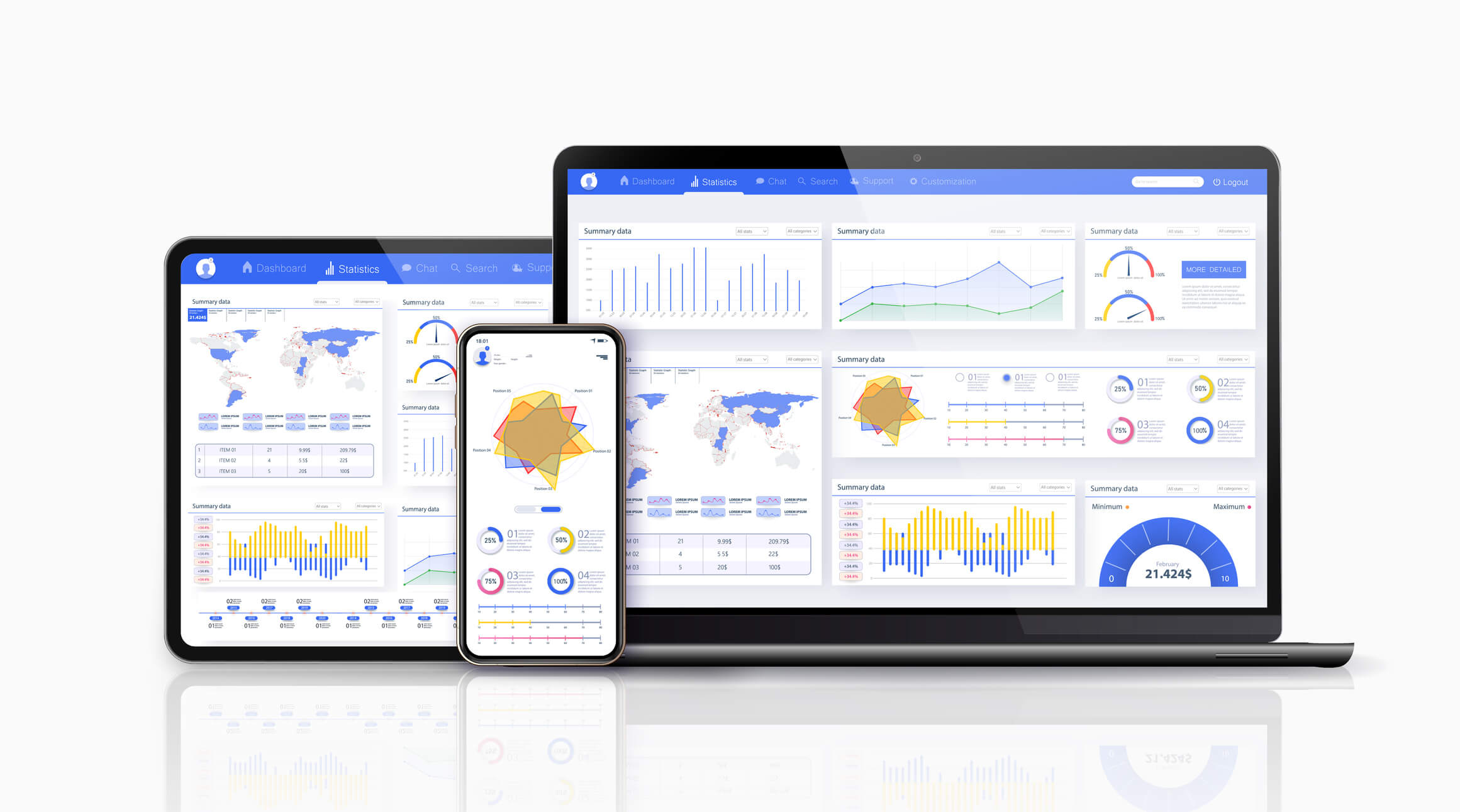# 10 Best Free Online Statistics Problem Solver 2022Acemyhomework BlogStatistics is a branch of Mathematics that teaches students how to make a graphical representation of mathematical information in a succinct manner. Statistics requires students to memorize many formulae to solve mathematical problems; hence, most students dread the topic. Thankfully, there are many online statistics problem solvers in 2022 that students can use to make their assignment easy.

Statistic questions can be demoralizing and get you to the point where you desperately stop to ask, "can someone answer my statistics question for free?" That should not always be the case. This list would be helpful to you if you did not grasp a concept or need help with your assignment.

It highlights statistics problem solvers and how helpful they would be to you. You no longer have to worry about cramming formulae. These applications and websites provide a solution to the questions.

#### 1. Stats solver

Stats solver helps you solve your statistics problems by providing a step-by-step guide and answers to your questions. For ease of access, Stats solver provides a list of topics such as numerical summary, basic probability, simple regression, to mention a few. Each topic gives a detailed overview of the concept and includes solved examples.

What is the website that solves any math problem? Stats solver is the best choice to consider. It provides a dialogue box for the user to input their problem and the function they wish to perform. It also has another dialogue box where the answer is output after the problem is solved. Stats solver also demonstrates the steps and formulae used while solving the problem.

#### 2. Mathway

Mathway is a free online statistics calculator that is easy to use while solving your statistics problems. It has clear symbols and interface, built-in examples and detailed instructions, making it beginner-friendly. The online app works as a:

• Ap statistics score calculator
• Probability calculator statistics
• T-test statistics calculator
• Percentile calculator
• Power calculator statistics

Can I use Mathway for statistics? As highlighted above, Mathway only computes linear regressions.

#### 3. Math10.com

Math10.com is a free and beginner-friendly statistics calculator. It is available online and performs functions such as calculating the following:

• Mean
• Mode
• Median
• Standard deviation

#### 4. Statistics Kingdom

Statistics Kingdom is a reliable online statistics math solver. It automatically checks assumptions, interprets results and outputs graphs and other charts. Depending on the type of function you want to perform, it has the following calculators:

• Test statistic calculators
• Regression calculator
• Sample size calculators
• Distribution calculators
• Statistics calculators

Each of these calculators has a step-by-step guide on using it.

#### 5. Stat trek

Stat trek provides the tools needed to solve statistics problems accurately. Apart from the detailed guide on learning statistics, the site has a stat toolbox that contains fast, accurate and user-friendly online calculators and statistical tables.

Stat trek is ideal for anyone looking to study advanced statistics.

#### 6. Calculator Soup

Calculator Soup is a beginner-friendly free online calculator and stats problems solver. Its statistics calculator allows you to perform functions such as:

• Basic statistics
• Descriptive statistics
• Interquartile range calculations
• Percentile calculations
• Quartile calculations
• Variance calculations
• Standard deviation calculations

#### 7. Calculator.net

Calculator.net is a beginner-friendly free statistics homework solver. It is a simple generalized statistics calculator that performs basic functions like calculating the following:

• Standard deviation
• Geometric mean
• Median
• Mode

It allows you to input your data and select the function you wish to perform.

#### 8. Statmagic

Statmagic is a statistics calculator app with 11 unique calculators that enable you to perform basic statistics functions. Statmagic has an automatic dataset import feature that works for iOS only. It allows the user to open a spreadsheet and copy the data onto the device's clipboard.

Statmagic allows you to perform the following functions:

• Descriptive Statistics
• The Normal Distribution
• Hypothesis Testing
• Confidence Intervals
• Regression

#### 9. Photomath

Photomath is the most-commonly used Mathematics education app. It is available for iOS only and allows users to perform basic arithmetic to advanced maths.

Photomath is free to use and gives a step-by-step explanation for every solution. Is there a Photomath for statistics? Statistics is one of the topics it covers.

But, can Photomath do statistics word problems? Yes. Photomath is one of the best apps to solve word problems. This makes it the most reliable app to refer to if you wish to pass your Math test.

#### 10. Social Science Statistics

Social Science Statistics provides free resources for students and researchers working with statistics in social sciences. Its tools have been designed with ease of use and clarity of presentation in mind.

The Social Science Statistics site has three main calculators:

• Statistical calculators. It deals with hypothesis testing.
• Descriptive statistics calculator. It has a standard deviation calculator, easy histogram, bear chart makers, and averages and variance calculators.
• P-value calculator. It allows you to derive the p-values from chi-square, Z and Pearson (r).

This list provides the best free online statistics problem solver in 2022. Most of these options guarantee the accuracy of their answers. However, some of them have limited functions. Nonetheless, that should not make you fret because Acemyhomework is your friend in need.

Acemyhomework is a community of reliable and qualified experts ready to help you with your homework, assignments, projects and essays. We are a globally recognized homework website that produces high-quality and plagiarism-free work within the set deadline. Our services guarantee better academic performance at a student-friendly rate. So, should you need a statistics math solver, we are one click away. You cn bank on our team’s expertise and professionalism.